# 1421 Square-shaped Sweet

This square-shaped puzzle is a Level 3 because you can start with clue 44 and work down the puzzle cell by cell to find the solution. You won’t get into a rhythm with this one, but logic will still help you to find the factors of the clues in order from top to bottom. That’s pretty sweet!Print the puzzles or type the solution in this excel file: 12 Factors 1419-1429

Now here are some sweet facts about the puzzle number 1421:

• 1421 is a composite number.
• Prime factorization: 1421 = 7 × 7 × 29, which can be written 1421 = 7² × 29.
• 1421 has at least one exponent greater than 1 in its prime factorization so √1421 can be simplified. Taking the factor pair from the factor pair table below with the largest square number factor, we get √1421 = (√49)(√29) = 7√29.
• The exponents in the prime factorization are 2 and 1. Adding one to each exponent and multiplying we get (2 + 1)(1 + 1) = 3 × 2 = 6. Therefore 1421 has exactly 6 factors.
• The factors of 1421 are outlined with their factor pair partners in the graphic below.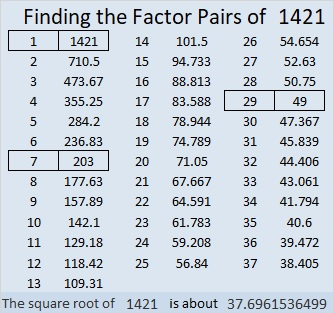1421 is also the hypotenuse of a Pythagorean triple:
980-1029-1421 which is (20-21-29) times 49.

# 1412 and Level 3

If you know the greatest common factor of 56 and 48, then you have taken the first step in solving this puzzle. Once you put the factors of 56 and 48 in the appropriate cells, work down from the top of the puzzle to the bottom, cell by cell, until you have put all the numbers from 1 to 10 in both the first column and the top row.Print the puzzles or type the solution in this excel file: 10 Factors 1410-1418

Here are a few facts about the puzzle number, 1412:

• 1412 is a composite number.
• Prime factorization: 1412 = 2 × 2 × 353, which can be written 1412 = 2² × 353.
• 1412 has at least one exponent greater than 1 in its prime factorization so √1412 can be simplified. Taking the factor pair from the factor pair table below with the largest square number factor, we get √1412 = (√4)(√353) = 2√353.
• The exponents in the prime factorization are 2 and 1. Adding one to each exponent and multiplying we get (2 + 1)(1 + 1) = 3 × 2 = 6. Therefore 1412 has exactly 6 factors.
• The factors of 1412 are outlined with their factor pair partners in the graphic below.1412 is the sum of two squares:
34² + 16² = 1412

1412 is the hypotenuse of a Pythagorean triple:
900-1088-1412 calculated from 34² – 16², 2(34)(16), 34² + 16²

# 1380 A Different Way to Look at the Logic

This puzzle looks a lot like puzzle #1378. The design is the same, but the clues are not in the same places. I made this puzzle to demonstrate that there is often more than one way to logically find all the factors of a puzzle. If it were a level 4 puzzle, the clues could be anywhere on the puzzle. But since it is a level 3 puzzle, start with the factors of 14 and 8, and then write the factors of 63 in the appropriate places. Continue with the clues in order from top to bottom until all the factors have been found.Print the puzzles or type the solution in this excel file: 10 Factors 1373-1388

Now I’ll share some information about the number 1380:

• 1380 is a composite number.
• Prime factorization: 1380 = 2 × 2 × 3 × 5 × 23, which can be written 1380 = 2² × 3 × 5 × 23
• 1380 has at least one exponent greater than 1 in its prime factorization so √1380 can be simplified. Taking the factor pair from the factor pair table below with the largest square number factor, we get √1380 = (√4)(√345) = 2√345
• The exponents in the prime factorization are 2, 1, 1, and 1. Adding one to each exponent and multiplying we get (2 + 1)(1 + 1)(1 + 1)(1 + 1) = 3 × 2 × 2 × 2 = 24. Therefore 1380 has exactly 24 factors.
• The factors of 1380 are outlined with their factor pair partners in the graphic below.There are MANY possible factor trees for 1380. Here’s one of them: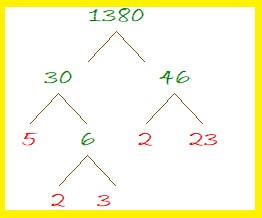1380 is the hypotenuse of a Pythagorean triple:
828-1104-1380 which is (3-4-5) times 276

# 1378 and Level 3

The first row with a clue has a 14 in it. Use that 14 and the clue in the same column, to figure out where to put one of the factor pairs of 14 in this puzzle.  Only use factor pairs where both numbers are from 1 to 10. Then work your way down the puzzle, row by row until you have found all the factors of this level 3 puzzle. The completed puzzle will look like a multiplication table with the factors in a different order.Print the puzzles or type the solution in this excel file: 10 Factors 1373-1388

Here is some information about the puzzle number, 1378:

• 1378 is a composite number.
• Prime factorization: 1378 = 2 × 13 × 53
• 1378 has no exponents greater than 1 in its prime factorization, so √1378 cannot be simplified.
• The exponents in the prime factorization are 1, 1, and 1. Adding one to each exponent and multiplying we get (1 + 1)(1 + 1)(1 + 1) = 2 × 2 × 2 = 8. Therefore 1378 has exactly 8 factors.
• The factors of 1378 are outlined with their factor pair partners in the graphic below.1378 is the 52nd triangular number because (52)(53)/2 = 1378.

1378 is the hypotenuse of FOUR Pythagorean triples:
222-1360-1378 which is 2 times (111-680-689)
530-1272-1378 which is (5-12-13) times 106
728-1170-1378 which is 26 times (28-45-53)
800-1122-1378 which is 2 times (400-561-689)

# 1359 and Level 3

The first row with a clue has a 6 in it. Use that 6 and another clue that goes with it, to figure out where to put the factors of 6 in this puzzle. Then work your way down the puzzle, row by row until you have found all the factors of this level 3 puzzle.Print the puzzles or type the solution in this excel file: 12 Factors 1357-1365

Here are a few facts about the number 1359:

• 1359 is a composite number.
• Prime factorization: 1359 = 3 × 3 × 151, which can be written 1359 = 3² × 151
• 1359 has at least one exponent greater than 1 in its prime factorization so √1359 can be simplified. Taking the factor pair from the factor pair table below with the largest square number factor, we get √1359 = (√9)(√151) = 3√151
• The exponents in the prime factorization are 2 and 1. Adding one to each exponent and multiplying we get (2 + 1)(1 + 1) = 3 × 2 = 6. Therefore 1359 has exactly 6 factors.
• The factors of 1359 are outlined with their factor pair partners in the graphic below.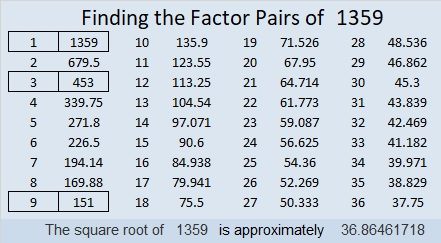1359 is the difference of two squares in three different ways:
680² – 679² = 1359
228² – 225² = 1359
80² – 71² = 1359

# 1352 and Level 3

If you know what numbers divide evenly into both 3 and 15, then you can solve this puzzle. Only use factor pairs where both numbers are from 1 to 10. Start at the top of the puzzle and work down cell by cell until you have found all the factors!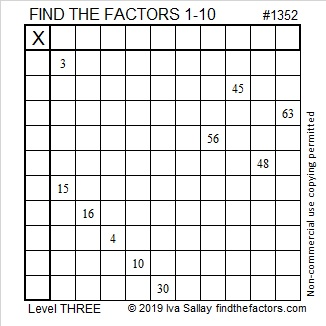Print the puzzles or type the solution in this excel file: 10 Factors 1347-1356

Here I explain how to solve the above puzzle:

Here are a few facts about the puzzle number, 1352:

• 1352 is a composite number.
• Prime factorization: 1352 = 2 × 2 × 2 × 11 × 11, which can be written 1352 = 2³ × 13²
• 1352 has at least one exponent greater than 1 in its prime factorization so √1352 can be simplified. Taking the factor pair from the factor pair table below with the largest square number factor, we get √1352 = (√676)(√2) = 26√2
• The exponents in the prime factorization are 3 and 2. Adding one to each exponent and multiplying we get (3 + 1)(2 + 1) = 4 × 3 = 12. Therefore 1352 has exactly 12 factors.
• The factors of 1352 are outlined with their factor pair partners in the graphic below.1352 is the hypotenuse of two Pythagorean triples:
520-1248-1352 which is (5-12-13) times 104
952-960-1352 which is 8 times (119-120-169)

# 1345 Level Three Puzzles Only

To solve a level 3 puzzle, start with a clue in the top row of the puzzle and find one other clue in the same row or column. Write the factors for those two clues in the appropriate cells and then work your way down the puzzle cell by cell until you have written the numbers from 1 to 10 in both the first column and the top row.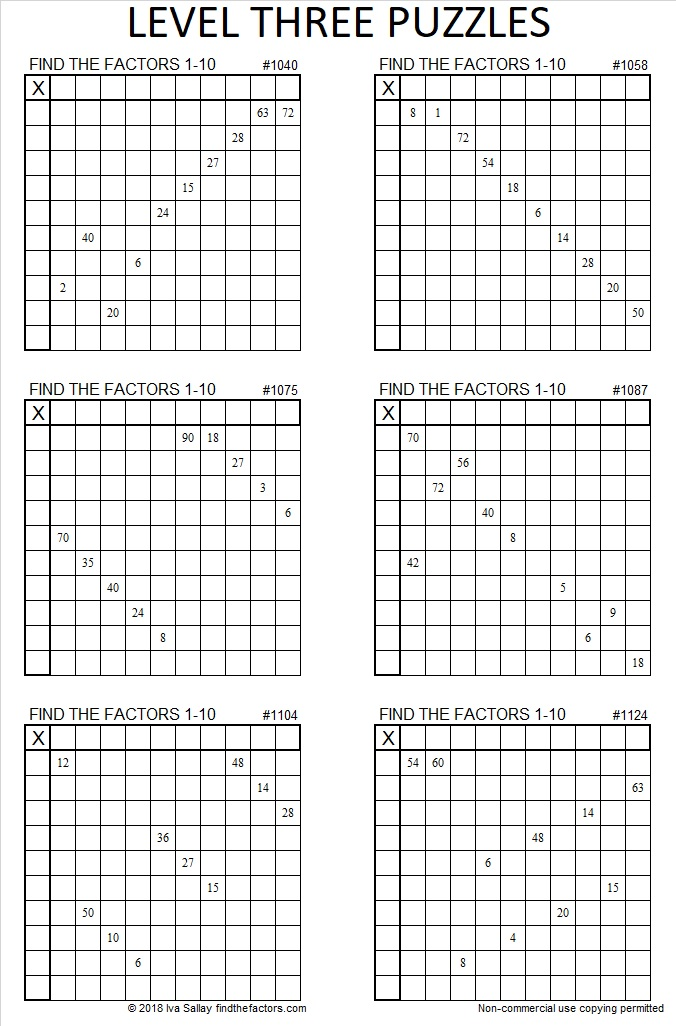Level 3 puzzles are a jump from level 2, but still significantly easier than any of the higher level puzzles, because the needed clues are kept in a logical order from the top of the puzzle to the bottom. You can print the puzzles above and work on them or you can access the whole collection in an excel sheet: Level 3’s from 2018. Print them or type the answers directly in excel.

Now I’ll share some information about the number 1345:

• 1345 is a composite number.
• Prime factorization: 1345 = 5 × 269
• 1345 has no exponents greater than 1 in its prime factorization, so √1345 cannot be simplified.
• The exponents in the prime factorization are 1, and 1. Adding one to each exponent and multiplying we get (1 + 1)(1 + 1) = 2 × 2 = 4. Therefore 1345 has exactly 4 factors.
• The factors of 1345 are outlined with their factor pair partners in the graphic below.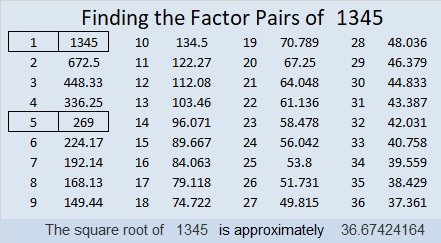1345 is the sum of two squares in two different ways:
36² + 7² = 1345
33² + 16² = 1345

1345 is also the hypotenuse of FOUR Pythagorean triples:
345-1300-1345 which is 5 times (69-260-269)
504-1247-1345 calculated from 2(36)(7), 36² – 7², 36² + 7²
807-1076-1345 which is (3-4-5) times 269
833-1056-1345 calculated from 33² – 16², 2(33)(16), 33² + 16²

# 1336 and Level 3

To solve this level 3 puzzle, start with the clue at the top of the grid, write the factors from 1 to 10 in the appropriate places, then do the same for each factor going down the puzzle row by row.Print the puzzles or type the solution in this excel file: 12 factors 1333-1341

Here’s some information about the puzzle number, 1336:

• 1336 is a composite number.
• Prime factorization: 1336 = 2 × 2 × 2 × 167, which can be written 1336 = 2³ × 167
• 1336 has at least one exponent greater than 1 in its prime factorization so √1336 can be simplified. Taking the factor pair from the factor pair table below with the largest square number factor, we get √1336 = (√4)(√334) = 2√334
• The exponents in the prime factorization are 3 and 1. Adding one to each and multiplying we get (3 + 1)(1 + 1) = 4 × 2 = 8. Therefore 1336 has exactly 8 factors.
• The factors of 1336 are outlined with their factor pairs in the graphic below.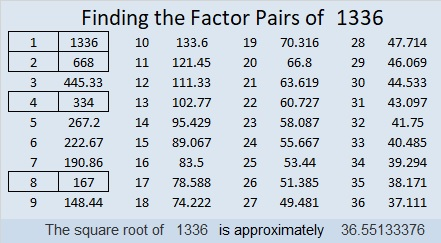1336 is the difference of two squares two ways:
335² – 333² = 1336
169² – 165² = 1336

# 1323 Christmas Tree

If you start at the top of this Christmas tree puzzle and work your way down, you can find all the factors that make this puzzle work like a multiplication table.

Print the puzzles or type the solution in this excel file:10-factors-1321-1332

Here are some facts about the number 1323:

• 1323 is a composite number.
• Prime factorization: 1323 = 3 × 3 × 3 × 7 × 7, which can be written 1323 = 3³ × 7²
• The exponents in the prime factorization are 3 and 2. Adding one to each and multiplying we get (3 + 1)(2 + 1) = 4 × 3 = 12. Therefore 1323 has exactly 12 factors.
• Factors of 1323: 1, 3, 7, 9, 21, 27, 49, 63, 147, 189, 441, 1323
• Factor pairs: 1323 = 1 × 1323, 3 × 441, 7 × 189, 9 × 147, 21 × 63, or 27 × 49
• Taking the factor pair with the largest square number factor, we get √1323 = (√441)(√3) = 21√3 ≈ 36.37307

Here is my favorite of the many possible factor trees for 1323:# 1313 Virgács and St. Nickolas Day

6 December is Saint Nickolas Day. Children in Hungary and other places in Europe wake up to find candy and virgács in their boots. You can read more about this wonderful tradition in Jön a Mikulás (Santa is Coming) or Die Feier des Weihnachtsmanns (The Celebration of Santa Claus). Today’s puzzle represents the virgács given to children who have been even the least bit naughty during the current year.

Print the puzzles or type the solution in this excel file: 12 factors 1311-1319

Now I’ll write a little bit about the number 1313:

• 1313 is a composite number.
• Prime factorization: 1313 = 13 × 101
• The exponents in the prime factorization are 1 and 1. Adding one to each and multiplying we get (1 + 1)(1 + 1) = 2 × 2 = 4. Therefore 1313 has exactly 4 factors.
• Factors of 1313: 1, 13, 101, 1313
• Factor pairs: 1313 = 1 × 1313 or 13 × 101
• 1313 has no square factors that allow its square root to be simplified. √1313 ≈ 36.23534

1313 is the sum of consecutive prime numbers in three different ways:
It is the sum of the twenty-one prime numbers from 19 to 107.
It is the sum of eleven consecutive primes:
97 + 101 + 103 + 107 + 109 + 113 + 127 + 131 + 137 + 139 + 149 = 1313,
and it is the sum of seven consecutive prime numbers:
173 + 179 + 181 + 191 + 193 + 197 + 199 = 1313

1313 is the sum of two squares two different ways:
32² + 17² = 1313
28² +  23² = 1313

1313 is the hypotenuse of FOUR Pythagorean triples:
255-1288-1313 calculated from 28² –  23², 2(28)(23), 28² +  23²
260-1287-1313 which is 13 times (20-99-101)
505-1212-1313 which is (5-12-13) times 101
735-1088-1313 calculated from 32² – 17², 2(32)(17), 32² + 17²